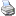# Elementary List Operationsbacklinks... CindyScript Control Operators Lists and Linear Algebra Table of Contents English 日本語

## Elementary List Operations

### Creating and Accessing Lists

#### Creating an integer sequence: `<int1>..<int2>`

Description: The expression `<int1>..<int2>` creates a list of consecutive integers starting with `<int1>` and ending with `<int2>`. If `<int1>` is larger than `<int2>`, then the empty list is returned.

 Code Result `4..9` `[4, 5, 6, 7, 8, 9]` `-2..2` `[-2, -1, 0, 1, 2]` `4..1` `[]`

#### The length of a list: `length(<list>)`

Description: This operator returns an integer that is equal to the number of elements in the `<list>`.

 Code Result `length([2 ,5 ,7 ,3])` `4` `length([2 ,[5, 4, 5] ,7 ,3]_2)` `3` `length(1..1000)` `1000`

Combining the `length` and the `repeat` operator allows one to list all elements of a list easily.

```repeat(length(list),
println(list_#);
)
```

One word of caution here: CindyScript is designed in such a way that it is seldom useful to traverse all the elements of a list using the `repeat` operator. There are more elegant ways.

#### Testing for containment: `contains(<list>,<expr>)`

Description: This operator returns either `true` or `false` depending on whether `<list>` contains the element <expr>.

 Code Result `contains([1,3,4,5],4)` `true` `contains([1,3,4,5],7)` `false` `contains([1,3,4,5],2*2)` `true`

### List Manipulation

#### Concatenation of lists: `concat(<list1>,<list2>)`

Description: This operator creates a list by concatenation of two other lists. This operator can equivalently be written as `<list1>++<list2>`.

 Code Result `concat(["a", "b"], ["c", "d"])` `["a", "b", "c", "d"]`

#### Removing elements from lists: `remove(<list1>,<list2>)`

Description: This operator creates a list by removing all elements that occur in `<list2>` from `<list1>`. This operator can equivalently be written as `<list1> -- <list2>`.

 Code Result `remove([1,3,4,5,1,5,6], [1,3,7])` `[4,5,5,6]` `[1,3,4,5,1,5,6]--[1,3,7]` `[4,5,5,6]`

#### Intersection of lists: `common(<list1>,<list2>)`

Description: This operator creates a list collecting all elements that are in both `<list1>` and `<list1>`. In the returned list the elements are sorted and each element occurs at most once. This operator can equivalently be written as `<list1>~~<list2>`.

 Code Result `common([1,3,4,5,1,5,6], [1,3,7])` `[1,3]` `[1,3,4,5,1,5,6]~~[1,3,7]` `[1,3]`

#### Appending an element: `append(<list>,<expr>)`

Description: This operator returns a list that is created by appending `<expr>` to the list `<list>` as its last element.
This operator can equivalently be written as `<list>:><expr>`.

 Code Result `append(["a", "b", "c"], "d")` `["a", "b", "c","d"]` `["a", "b", "c"]:>"d"` `["a", "b", "c","d"]`

#### Prepending an element: `prepend(<expr>,<list>)`

Description: This operator returns a list that is created by prepending `<expr>` to the list `<list>` as its first element.
This operator can equivalently be written as `<expr><:<list>`.

 Code Result `prepend("d",["a", "b", "c"])` `["d","a", "b", "c"]` `"d"<:["a", "b", "c"]` `["d","a", "b", "c"]`

### Traversing Lists

#### The forall loop: `forall(<list>,<expr>)`

Description: This operator is useful for applying an operation to all elements of a list. It takes a `<list>` as first argument. It produces a loop in which `<expr>` is evaluated for each entry of the list. For each run, the run variable `#` takes the value of the corresponding list entry.

Example:

```a=["this","is","a","list"];
forall(a,println(#))
```

This code fragment produces the output

```this
is
a
list
```

#### The forall loop: `forall(<list>,<var>,<expr>)`

Description: Similar to `forall(<list>,<expr>)`, but the run variable is now named `<var>`.

#### Applying an expression: `apply(<list>,<expr>)`

Description: This operator generates a new list by applying the operation `<expr>` to all elements of a list and collecting the results. As usual, `#` is the run variable, which successively takes the value of each element in the list.

 Code Result `apply([1, 2, 3, 4, 5],#^2)` `[1, 4, 9, 16, 25]` `apply([1, 2, 3, 4, 5],#+5)` `[6, 7, 8, 9, 10]` `apply(1..5, [#,#^2])` `[[1, 1], [2, 4], [3, 9], [4, 16], [5, 25]]`

#### Applying an expression: `apply(<list>,<var>,<expr>)`

Description: Similar to `apply(<list>,<expr>)`, but the run variable is now named `<var>`.

#### Selecting elements of a list: `select(<list>,<boolexpr>)`

Description: This operator selects all elements of a list for which a certain condition is satisfied. The condition is supposed to be encoded by `<boolexpr>`. This expression is assumed to return a `<bool>` value. As usual, `#` is the run variable, which successively take the value of all elements in the list.

 Code Result `select(1..10, isodd(#))` `[1, 3, 5, 7, 9]` `select(0..10, #+# == #^2)` `[0,2]`

A high-level application of the `select` operator is given by the following example:

```divisors(x):=select(1..x,mod(x,#)==0);
primes(n):=select(1..n,length(divisors(#))==2);
println(primes(100))
```

It produces the output

```[2,3,5,7,11,13,17,19,23,29,31,37,41,43,47,53,59,61,67,71,73,79,83,89,97]
```

In this example, first a function `divisors(x)` is defined by selecting those numbers that divide `x` without any remainder. Then a function `primes(n)` is defined that selects all numbers between `1` and `n` that have exactly two divisors. These numbers are the primes.

#### Selecting elements of a list: `select(<list>,<var>,<boolexpr>)`

Description: Similar to `select(<list>,<boolexpr>)`, but the run variable is now named <var>.